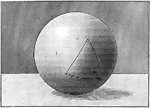### Isosceles Spherical Triangle

Illustration of an isosceles spherical triangle.### Isosceles Spherical Triangle

Illustration of an isosceles spherical triangle.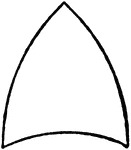### Spherical Triangle

Diagram showing a spherical triangle.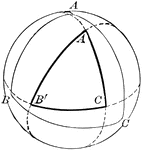### Spherical Triangle

Diagram showing a spherical triangle constructed by the intersection of polar arcs.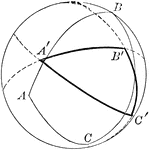### Spherical Triangle

Diagram showing a spherical triangle constructed by the intersection of polar arcs.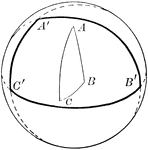### Spherical Triangle

Diagram showing a spherical triangle constructed by the intersection of polar arcs.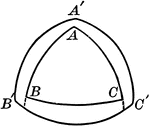### Polar and Spherical Triangles

Illustration of polar and spherical triangles.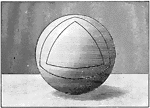### Polar Triangles in Sphere

Illustration of polar triangles.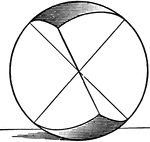### Symmetric Spherical Triangles

Illustration of symmetrical spherical triangles.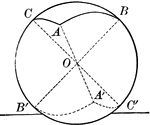### Symmetric Spherical Triangles

Illustration of symmetrical spherical triangles.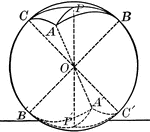### Symmetric Spherical Triangles

Illustration of symmetrical spherical triangles.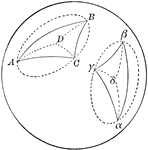### Two Symmetrical Spherical Triangles

Diagram showing a 2 symmetrical spherical triangles.